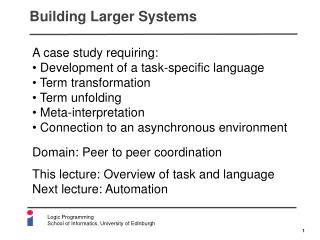Download PresentationBuilding Larger SystemsBuilding Larger Systems - PowerPoint PPT Presentation

Download PresentationBuilding Larger Systems
An Image/Link below is provided (as is) to download presentation

Download Policy: Content on the Website is provided to you AS IS for your information and personal use and may not be sold / licensed / shared on other websites without getting consent from its author. While downloading, if for some reason you are not able to download a presentation, the publisher may have deleted the file from their server.

- - - - - - - - - - - - - - - - - - - - - - - - - - - E N D - - - - - - - - - - - - - - - - - - - - - - - - - - -
Presentation Transcript

1. Building Larger Systems • A case study requiring: • Development of a task-specific language • Term transformation • Term unfolding • Meta-interpretation • Connection to an asynchronous environment Domain: Peer to peer coordination This lecture: Overview of task and language Next lecture: Automation

2. Overview Constraint solver Protocol interpreter Transport on media Media Agent a2 Agent a1 out in Global state of this interaction

3. Basic Example Agent a2 ask(p(Y)) Agent a1 tell(p(a)) know(p(a)) ask(q(Y)) Agent a3 query_from(p(Y), a2) query_from(q(Y), a3)

4. Example Protocol Role Message out A requester will ask about something from an informerthen get an answer from it then continue as a requester An informer will be asked by a requester then should tell the requester if it knows a(requester, A) ::= ask(X) => a(informer, B)  query_from(X, B) then tell(X) <= a(informer, B) then a(requester, A) a(informer, B) ::= ask(X) <= a(requester, A) then tell(X) => a(requester, A)  know(X) Constraint Message in Recursive role

5. Operator Declarations for Protocol :- op(900, xfx, '::='), op(800, xfx, '=>'), op(800, xfx, '<='), op(830, xfx, ‘'), op(820, xfy, and), op(850, xfy, par), op(850, xfy, then), op(850, xfy, or). ::= a(informer, B) then <=  ask(X) a(requester, A) know(X) => a(informer, B) ::= ask(X) <= a(requester, A) then tell(X) => a(requester, A)  know(X) tell(X) a(requester, A)

6. Interaction Example General Specific a(requester, A) ::= ask(X) => a(informer, B)  query_from(X, B) then tell(X) <= a(informer, B) then a(requester, A) a(informer, B) ::= ask(X) <= a(requester, A) then tell(X) => a(requester, B)  know(X) a(requester, a1) ::= ask(p(Y)) => a(informer, a2) then tell(p(Y)) <= a(informer, a2) then a(requester, a1) Agent a1 Agent a2 ask(p(Y)) query_from(p(Y), a2)

7. Interaction Example Specific General a(requester, A) ::= ask(X) => a(informer, B)  query_from(X, B) then tell(X) <= a(informer, B) then a(requester, A) a(informer, B) ::= ask(X) <= a(requester, A) then tell(X) => a(requester, B)  know(X) a(requester, a1) ::= ask(p(Y)) => a(informer, a2) then tell(p(Y)) <= a(informer, a2) then a(requester, a1) a(informer, a2) ::= ask(p(Y)) <= a(requester, a1) then tell(p(Y)) => a(requester, a1)  know(p(Y)) Agent a1 Agent a2 ask(p(Y))

8. Interaction Example General Specific a(requester, A) ::= ask(X) => a(informer, B)  query_from(X, B) then tell(X) <= a(informer, B) then a(requester, A) a(informer, B) ::= ask(X) <= a(requester, A) then tell(X) => a(requester, B)  know(X) a(requester, a1) ::= ask(p(Y)) => a(informer, a2) then tell(p(Y)) <= a(informer, a2) then a(requester, a1) a(informer, a2) ::= ask(p(a)) <= a(requester, a1) then tell(p(a)) => a(requester, a1) Agent a1 Agent a2 tell(p(a)) know(p(a))

9. Interaction Example Specific General a(requester, A) ::= ask(X) => a(informer, B)  query_from(X, B) then tell(X) <= a(informer, B) then a(requester, A) a(informer, B) ::= ask(X) <= a(requester, A) then tell(X) => a(requester, B)  know(X) a(requester, a1) ::= ask(p(a)) => a(informer, a2) then tell(p(a)) <= a(informer, a2) then a(requester, a1) ::= ask(X) => a(informer, B)  query_from(X, B) then tell(X) <= a(informer, B) then a(requester, a1) a(informer, a2) ::= ask(p(a)) <= a(requester, a1) then tell(p(a)) => a(requester, a1) Agent a1 Agent a2 tell(p(a))

10. And so on… Specific General a(requester, A) ::= ask(X) => a(informer, B)  need(X) and source(B) then tell(X) <= a(informer, B) then a(requester, A) a(informer, B) ::= ask(X) <= a(requester, A) then tell(X) => a(requester, B)  know(X) a(requester, a1) ::= ask(p(a)) => a(informer, a2) then tell(p(a)) <= a(informer, a2) then a(requester, a1) ::= ask(q(Y)) => a(informer, a3) then tell(q(Y)) <= a(informer, a3) then a(requester, a1) a(informer, a2) ::= ask(p(a)) <= a(requester, a1) then tell(p(a)) => a(requester, a1) Agent a2 Agent a1 Agent a3 q(Y) query_from(q(Y), a3)

11. Agents Determining Peers Finder Person Inform his/her agent Available? Organiser Ask for agent of a person Confirm/disconfirm Suggest best people Ask for best people Headhunter

12. For Example… Agent is m_wooldridge Person Finder n_jennings d_sleeman Agent for mike_wooldridge? Available? Confirm Organiser d_robertson Person m_wooldridge Agent is n_jennings Available? Agent for nick_jennings? Confirm Ask for best people Suggest [nick_jennings,mike_wooldridge] Headhunter w_hall DEMO

13. Protocol for Example a(organiser(Topic,H,F), O) ::= ask(best_people(Topic)) => a(headhunter, H) then inform(best_people(Topic, People)) <= a(headhunter, H) then a(locator(F,People,Locations), O) then a(time_coordinator(People,Locations,Topic,Times,Time), O)  times(Times) a(locator(F,People,Locations), L) ::= ( ask(locate(Person)) => a(finder, F)  People = [Person|Rp] and Locations = [Loc|Rl] then inform(located(Person, Loc)) <= a(finder, F) then a(locator(F,Rp, Rl), L) ) or null  People = [] and Locations = [] An organiser service on some topic will ask a headhunter service for the best people on that topic, then it will be informed by the headhunter who those people are, then it will change role to be a locator for these people, then it will become a time coordinator for these people A locator for a set of people will ask a finder service for an address for a person in the set, then it will be informed by the finder of the address, then it will continue to locate all the other people in the set until it finishes at the end